This post will guide you how to use Google Sheets CEILING function with syntax and examples.

## Description

The Google Sheets CEILING function returns a given number rounded up to the nearest multiple of a given number of significance. You can use the CEILING function to round up a number to the nearest multiple of a given number.

The CEILING function is a build-in function in Google Sheets and it is categorized as a MATH function.

## Syntax

The syntax of the CEILING function is as below:

= CEILING (number, significance)

Where the CEILING function arguments are:

• number – This is a required argument. The number that you want to round up.
• significance – This is a required argument. The multiple of significance to which you want to round a number to.

Note:

• If either number and significance arguments are non-numeric, the CEILING function will return the #VALUE! Error.
• If the number argument is negative, and the significance argument is also negative, the number is rounded down, and it will be away from 0.
• If the number argument is negative and the significance is positive, the number is rounded up towards zero.

## Google Sheets CEILING Function Examples

The below examples will show you how to use google sheets CEILING Function to round a number up to nearest multiple.

1# to round 4.6 up to nearest multiple of 3, enter the following formula in Cell B1.

`=CEILING(4.6,3)`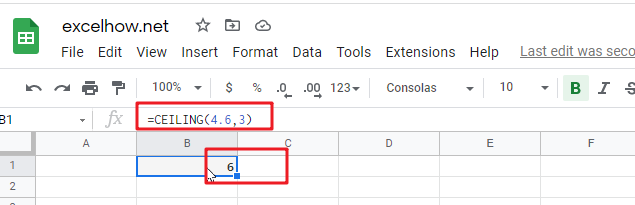2# to round -4.6 up to nearest multiple of -3, enter the following formula in Cell B2.

`=CEILING(-4.6,-3)`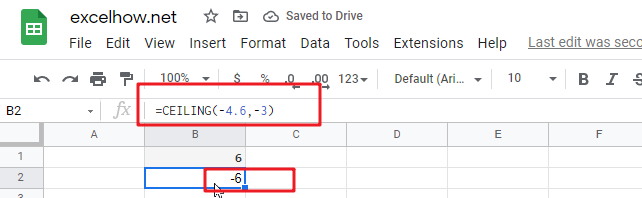3# to round -4.6 up to nearest multiple of 2, enter the following formula in Cell B3.

`=CEILING(-4.6,2)`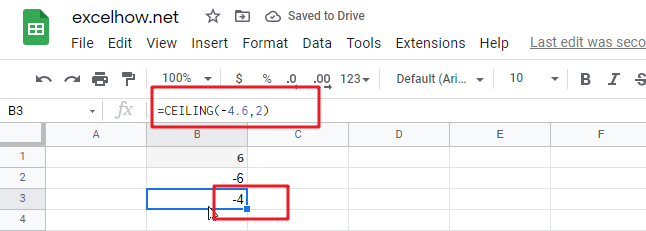4# to round 2.6 up to nearest multiple of 0.2, enter the following formula in Cell B4.

`=CEILING(2.6,0.2)`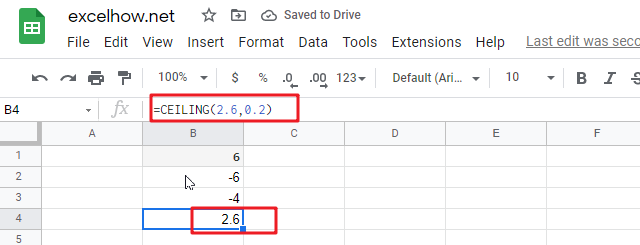5# to round 0.26 up to nearest multiple of 0.02, enter the following formula in Cell B5.

`=CEILING(0.26,0.02)`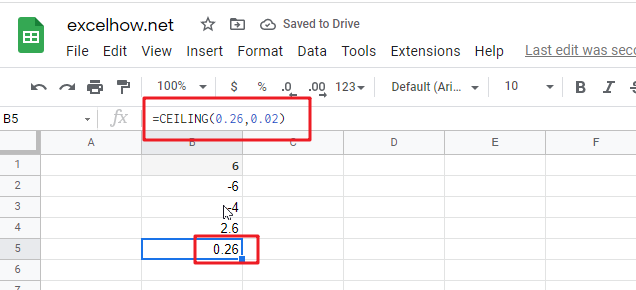Related Posts

This post will guide you how to use Google Sheets LN function with syntax and examples. Description The Google Sheets LN function returns the natural logarithm of a given number.  And the natural logarithms are based on the constant e ...

This post will guide you how to use Google Sheets INT function with syntax and examples. Description The Google Sheets INT function returns the integer portion of a given number. And it will round a given number down to the ...

This post will guide you how to use Google Sheets FLOOR function with syntax and examples. Description The Google Sheets FLOOR function returns a number rounded down to the nearest multiple of significance. So it will return a rounded number. ...

This post will guide you how to use Google Sheets EXP function with syntax and examples. Description The Google Sheets EXP function returns e raised to the power of a number.  The constant e is equal to 2.71828182845904, and it ...

This post will guide you how to use Google Sheets FACT function with syntax and examples. Description The Google Sheets FACT function returns the factorial of a given number.  The factorial of a given number is equal to 1*2*3…* number. ...

This post will guide you how to use Google Sheets EVEN function with syntax and examples. Description The Google Sheets EVEN function rounds a given number up to the nearest even integer. You can use the EVEN function to return ...

This post will guide you how to use Google Sheets COUNTBLANK function with syntax and examples. Description The Google Sheets COUNTBLANK function use to count the number of empty cells in a range of cells. You can use the COUNTBLANK ...